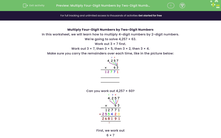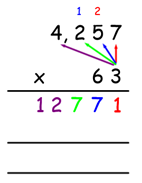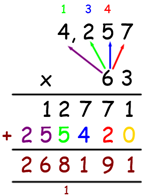# Multiply Four-Digit Numbers by Two-Digit Numbers

In this activity, students will learn how to multiply 4-digit numbers by 2-digit numbers.Key stage:  KS 2

Curriculum topic:   Number: Addition, Subtraction, Multiplication and Division

Curriculum subtopic:   Multiply to Four Digits (Long Multiplication)

Popular topics:   Multiplication worksheets

Difficulty level:#### Worksheet Overview

Multiply Four-Digit Numbers by Two-Digit Numbers

In this worksheet, we will learn how to multiply 4-digit numbers by 2-digit numbers.

We're going to solve 4,257 × 63.

Work out 3 × 7 first.

Work out 3 × 7, then 3 × 5, then 3 × 2, then 3 × 4.

Make sure you carry the remainders over each time, like in the picture below:Can you work out 4,257 × 60?First, we work out

6 × 7

6 × 5

6 × 2

6 × 4

adding on the numbers you carry over.

Finally, add the two numbers together: 12771 + 255420 = 268191.

Now, let's put this method to the test!

### What is EdPlace?

We're your National Curriculum aligned online education content provider helping each child succeed in English, maths and science from year 1 to GCSE. With an EdPlace account you’ll be able to track and measure progress, helping each child achieve their best. We build confidence and attainment by personalising each child’s learning at a level that suits them.

Get started